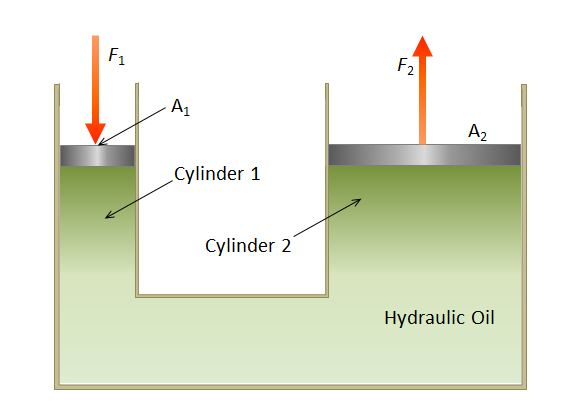# Hydraulic SystemA hydraulic drive system is a drive or transmission system that uses pressurized hydraulic fluid to drive hydraulic machinery. The term hydrostatic refers to the transfer of energy from flow and pressure, not from the kinetic energy of the flow. The above shows a hydraulic system where the piston area $A_2$ is $10$ times larger than the piston area $A_1$. If we denote the magnitudes of the forces acting on cylinder $1$ and cylinder $2$ as $F_1$ and $F_2,$ respectively, what is the ratio $F_1:F_2?$

×